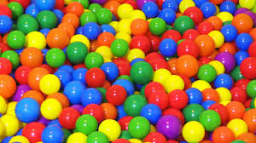# Colored balls

There are colored balls in the bag. One third of them are blue, one sixth is green, five twelfths are yellow and the remaining 10 balls are red. How many yellow balls are in the bag?

x =  50

### Step-by-step explanation:Did you find an error or inaccuracy? Feel free to write us. Thank you!

Tips for related online calculators
Need help calculating sum, simplifying, or multiplying fractions? Try our fraction calculator.
Do you have a linear equation or system of equations and looking for its solution? Or do you have a quadratic equation?